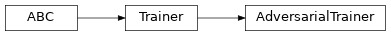Inheritance Diagramclass ashpy.trainers.AdversarialTrainer(generator, discriminator, generator_optimizer, discriminator_optimizer, generator_loss, discriminator_loss, epochs, metrics=None, callbacks=None, logdir=PosixPath('/home/docs/checkouts/readthedocs.org/user_builds/ashpy/checkouts/latest/docs/source/log'), log_eval_mode=<LogEvalMode.TEST: 1>, global_step=None)[source]

Primitive Trainer for GANs subclassed from ashpy.trainers.Trainer.

Examples

import shutil
import operator

generator = models.gans.ConvGenerator(
layer_spec_input_res=(7, 7),
layer_spec_target_res=(28, 28),
kernel_size=(5, 5),
initial_filters=32,
filters_cap=16,
channels=1,
)

discriminator = models.gans.ConvDiscriminator(
layer_spec_input_res=(28, 28),
layer_spec_target_res=(7, 7),
kernel_size=(5, 5),
initial_filters=16,
filters_cap=32,
output_shape=1,
)

# Losses
generator_bce = losses.gan.GeneratorBCE()
minmax = losses.gan.DiscriminatorMinMax()

# Real data
batch_size = 2
mnist_x, mnist_y = tf.zeros((100,28,28)), tf.zeros((100,))

# Trainer
epochs = 2
metrics = [
metrics.gan.InceptionScore(
# Fake inception model
models.gans.ConvDiscriminator(
layer_spec_input_res=(299, 299),
layer_spec_target_res=(7, 7),
kernel_size=(5, 5),
initial_filters=16,
filters_cap=32,
output_shape=10,
),
model_selection_operator=operator.gt,
)
]
generator=generator,
discriminator=discriminator,
generator_loss=generator_bce,
discriminator_loss=minmax,
epochs=epochs,
metrics=metrics,
logdir=logdir,
)

# take only 2 samples to speed up tests
real_data = (
tf.data.Dataset.from_tensor_slices(
(tf.expand_dims(mnist_x, -1), tf.expand_dims(mnist_y, -1))).take(batch_size)
.batch(batch_size)
.prefetch(1)
)

# Add noise in the same dataset, just by mapping.
# The return type of the dataset must be: tuple(tuple(a,b), noise)
dataset = real_data.map(
lambda x, y: ((x, y), tf.random.normal(shape=(batch_size, 100)))
)

trainer(dataset)
shutil.rmtree(logdir)

Initializing checkpoint.
Starting epoch 1.
Epoch 1 completed.
Starting epoch 2.
Epoch 2 completed.
Training finished after 2 epochs.


Methods

 __init__(generator, discriminator, …[, …]) Instantiate a AdversarialTrainer. call(dataset[, log_freq, …]) Perform the adversarial training. train_step(real_xy, g_inputs) Train step for the AdversarialTrainer.

Attributes

 ckpt_id_callbacks ckpt_id_discriminator ckpt_id_generator ckpt_id_global_step ckpt_id_optimizer_discriminator ckpt_id_optimizer_generator ckpt_id_steps_per_epoch context Return the training context.
__init__(generator, discriminator, generator_optimizer, discriminator_optimizer, generator_loss, discriminator_loss, epochs, metrics=None, callbacks=None, logdir=PosixPath('/home/docs/checkouts/readthedocs.org/user_builds/ashpy/checkouts/latest/docs/source/log'), log_eval_mode=<LogEvalMode.TEST: 1>, global_step=None)[source]

Instantiate a AdversarialTrainer.

Parameters: generator (tf.keras.Model) – A tf.keras.Model describing the Generator part of a GAN. discriminator (tf.keras.Model) – A tf.keras.Model describing the Discriminator part of a GAN. generator_optimizer (tf.optimizers.Optimizer) – A tf.optimizers to use for the Generator. discriminator_optimizer (tf.optimizers.Optimizer) – A tf.optimizers to use for the Discriminator. generator_loss (ashpy.losses.executor.Executor) – A ash Executor to compute the loss of the Generator. discriminator_loss (ashpy.losses.executor.Executor) – A ash Executor to compute the loss of the Discriminator. epochs (int) – number of training epochs. metrics (Union[Tuple[Metric], List[Metric], None]) – (List): list of ashpy.metrics.Metric to measure on training and validation data. callbacks (List) – list of ashpy.callbacks.Callback to measure on training and validation data. logdir (Union[Path, str]) – checkpoint and log directory. log_eval_mode (LogEvalMode) – models’ mode to use when evaluating and logging. global_step (Optional[tf.Variable]) – tf.Variable that keeps track of the training steps. None
_build_and_restore_models(dataset)[source]

Build and restore a Subclassed model by firstly calling it on some data.

_train_step[source]

Training step with the distribution strategy.

call(dataset, log_freq=10, measure_performance_freq=10)[source]

Parameters: dataset (tf.data.Dataset) – The adversarial training dataset. log_freq (int) – Specifies how many steps to run before logging the losses, e.g. log_frequency=10 logs every 10 steps of training. Pass log_frequency<=0 in case you don’t want to log. measure_performance_freq (int) – Specifies how many steps to run before measuring the performance, e.g. measure_performance_freq=10 measures performance every 10 steps of training. Pass measure_performance_freq<=0 in case you don’t want to measure performance.
train_step(real_xy, g_inputs)[source]